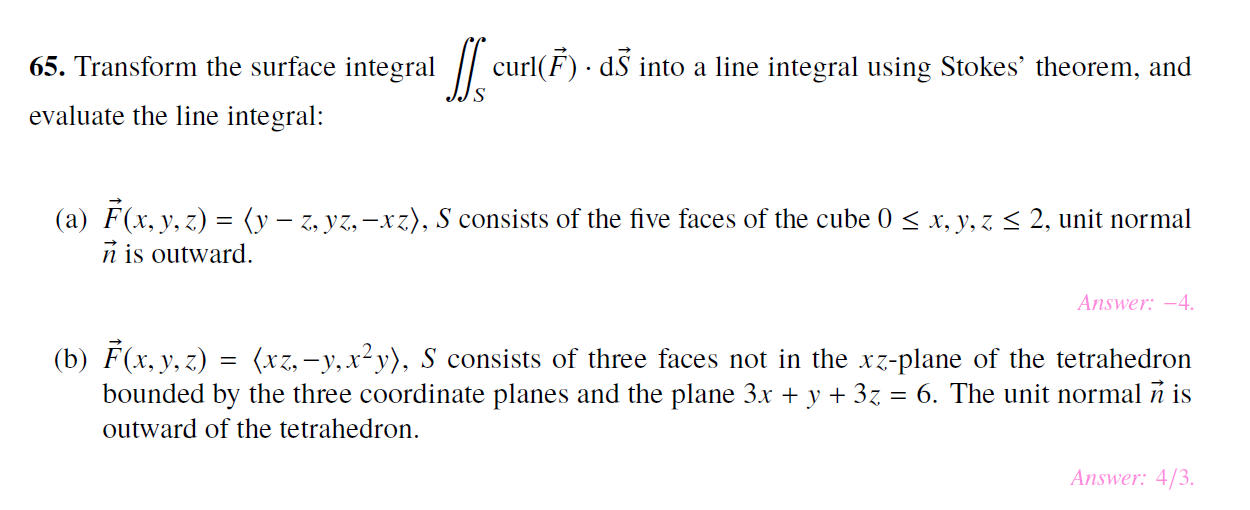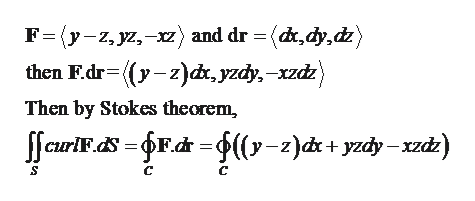# 65. Transform the surface integral curl(F dS into a line integral using Stokes' theorem, andevaluate the line integral:(a) F(x, y, z(y-, yz,-xz), S consists of the five faces of the cube 0n is outward2, unit normalx, y, zAnswer: -4(b) F(x, y, z) (xz,-y,x2y), S consists of three faces not in the xz-plane of the tetrahedronbounded by the three coordinate planes and the plane 3.x + y + 3z = 6. The unit normal n isoutward of the tetrahedron.Answer: 4/3

Question
44 viewshelp_outlineImage Transcriptionclose65. Transform the surface integral curl(F dS into a line integral using Stokes' theorem, and evaluate the line integral: (a) F(x, y, z(y-, yz,-xz), S consists of the five faces of the cube 0 n is outward 2, unit normal x, y, z Answer: -4 (b) F(x, y, z) (xz,-y,x2y), S consists of three faces not in the xz-plane of the tetrahedron bounded by the three coordinate planes and the plane 3.x + y + 3z = 6. The unit normal n is outward of the tetrahedron. Answer: 4/3 fullscreen
check_circle

star
star
star
star
star
2 Ratings
Step 1

We’ll answer the first question since the exact one wasn’t specified. Please submit a new question specifying the one you’d like answered.

Stokes theorem – If S be a smooth surface which is bounded by a simple, closed and smooth boundary curve, then

Step 2

(a)Consider the given function and the surface as,

Step 3

Applying stokes theorem on t...help_outlineImage TranscriptioncloseF=y-z,yz,-z) and dr = (dk,dy,dz) then F.dr ((y-z)dr, yzcy, xzdz) Then by Stokes theorem CrIdsFdr=fl(y-z)dk+yzdy -xzdz) C fullscreen

### Want to see the full answer?

See Solution

#### Want to see this answer and more?

Solutions are written by subject experts who are available 24/7. Questions are typically answered within 1 hour.*

See Solution
*Response times may vary by subject and question.
Tagged in

### Math